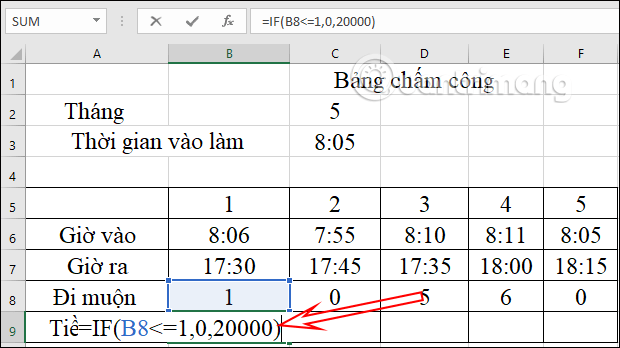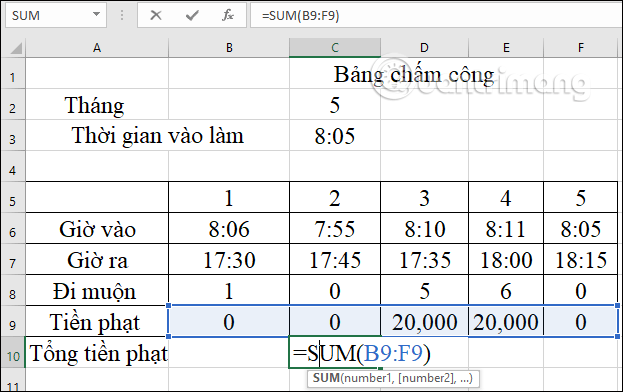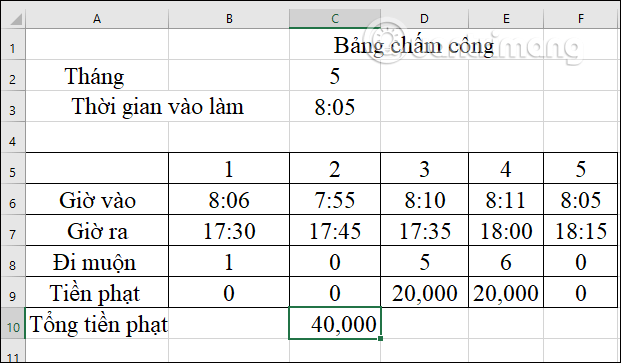# How to calculate late fines in Excel

283 total views

2021-06-07 20:07:21

We can create a data table in Excel that reports the number of minutes an employee is late to work and from there can calculate the amount of late penalty that the employee or student has to pay. This makes the calculation and statistics of the human resources department in the company, in the class or in the group made simpler and easier, instead of having to manually calculate it. The following article will guide you how to calculate the number of minutes late in Excel.

## Instructions for calculating the amount of late arrivals in Excel

Step 1:

First of all, we proceed to enter the date and time of going to work, and the official entry time, or a person’s time of school into Excel as below.

Step 2:

Now you will need Calculate the number of minutes that an employee is late to work that by using the MINUTE function in Excel combined with the IF function in Excel.

You enter the formula as below.

``=MINUTE(IF(B6>\$C\$3,B6-\$C\$3,0))``

That is, if the timekeeping time is larger than the fixed entry time, it will be subtracted to get the number of minutes late to work. You press Enter to get the number of minutes late to work.Step 3:

Dragging the result box to the side, the remaining cells will get the number of minutes late to work of the remaining days if any.Step 4:

Next below will Enter the dates of the fine for being late for work. Regulation example If the number of minutes late to work is less than or equal to 1 minute, there will be no fine of 20,000 VND. If more than 1 minute will be charged 20,000 VND.

We enter the formula as below:

``=IF(B8<=1,0,20000)``Pressing Enter will output whether the date will be fined or not. You drag the results to the remaining cells to see the fines for other late days.Step 5:

In the end we will calculate the total amount of fines that the person has to close when he is late for work with the SUM function in Excel.So we will know the total amount of fines that the employee will have to pay..

#calculate #late #fines #Excel

Share